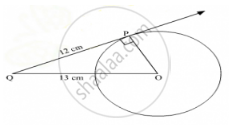# From a Point Q, 13 Cm Away from the Centre of a Circle, the Length of Tangent Pq to the Circle is 12 Cm. the Radius of the Circle (In Cm) is - Mathematics

MCQ

From a point Q, 13 cm away from the centre of a circle, the length of tangent PQ to the circle is 12 cm. The radius of the circle (in cm) is

#### Options

• A. 25

• B. sqrt313

• C. 5

• D. 1

#### Solution

The given information can be represented diagrammatically as follows:Let O be the centre of the circle.

Given: PQ = 12 cm and OQ = 13 cm.

To find: Radius of the circle

PQ is a tangent drawn from the external point Q to the circle.

∠ OPQ = 90° (Radius is perpendicular to the tangent at the point of contact)

On applying Pythagoras theorem in ΔOPQ, we obtain:

OQ2 = OP2 + PQ2

∴ OP2 = OQ2 − PQ2

⇒ OP2 = (13 cm)2 − (12 cm)2

⇒ OP2 = 169cm2 − 144 cm2

⇒ OP= 25 cm2

⇒ OP = 5 cm

Thus, the radius of circle is 5 cm.

Hence, the correct answer is C

Is there an error in this question or solution?
2011-2012 (March) All India Set 1

Share